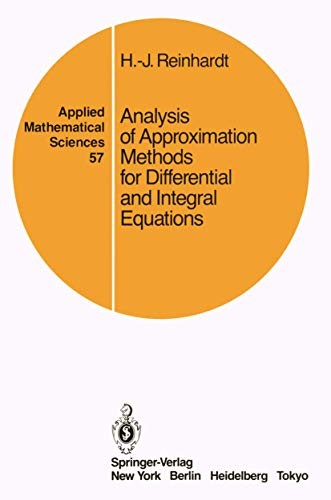# Analysis of Approximation Methods for Differential and Integral Equations by Hans-Jurgen Reinhardt

In Stock
£11.99
The text treats numerous methods for approximating solutions of three classes of problems: (elliptic) boundary-value problems, (hyperbolic and parabolic) initial value problems in partial differential equations, and integral equations of the second kind.
Only 1 left

## Analysis of Approximation Methods for Differential and Integral Equations Summary

### Analysis of Approximation Methods for Differential and Integral Equations by Hans-Jurgen Reinhardt

This book is primarily based on the research done by the Numerical Analysis Group at the Goethe-Universitat in Frankfurt/Main, and on material presented in several graduate courses by the author between 1977 and 1981. It is hoped that the text will be useful for graduate students and for scientists interested in studying a fundamental theoretical analysis of numerical methods along with its application to the most diverse classes of differential and integral equations. The text treats numerous methods for approximating solutions of three classes of problems: (elliptic) boundary-value problems, (hyperbolic and parabolic) initial value problems in partial differential equations, and integral equations of the second kind. The aim is to develop a unifying convergence theory, and thereby prove the convergence of, as well as provide error estimates for, the approximations generated by specific numerical methods. The schemes for numerically solving boundary-value problems are additionally divided into the two categories of finite difference methods and of projection methods for approximating their variational formulations.

### Why buy from World of BooksOur excellent value books literally don't cost the earthFree delivery in the UKEvery used book bought is one saved from landfill

I: Presentation of Numerical Methods.- 1. Finite-Difference Methods for Boundary-Value Problems.- 1.1. Sample Problems.- 1.2. Finite-Difference Methods for Linear, Second Order Ordinary Differential Equations.- 1.3. A Finite-Difference Approximation for the Cantilevered Beam Problem.- 1.4. Finite-Difference Methods for a Nonlinear Boundary-Value Problem.- 1.5. Finite-Difference Approximations for Two-Dimensional Elliptic Equations.- References.- 2. Projection Methods for Variational Equations.- 2.1. Basic Properties of Variational Equations and Sample Problems.- 2.2. Sample Problems (Revised).- 2.3. The Ritz Method.- 2.4. Galerkin Methods and the Method of Least Squares.- 2.5. Projection Methods for Nonlinear Problems.- References.- 3. Approximation Methods for Integral Equations of the Second Kind.- 3.1. Linear Integral Equations of the Second Kind.- 3.2. Quadrature Methods.- 3.3. Projection Methods.- 3.4. Approximations of Nonlinear Integral Equations.- References.- 4. Approximation Methods for Initial Value Problems in Partial Differential Equations 74.- 4.1. Difference Methods for the Heat Equation.- 4.2. Galerkin Methods for the Heat Equation.- 4.3. Numerical Methods for the Wave Equation.- 4.4. The Numerical Solution of Nonlinear Initial Value Problems.- 4.5. Pure Initial Value Problems and a General Representation of Approximation Methods.- References.- II: Convergence Theory.- 5. The Concepts of Discrete Convergence and Discrete Approximations.- 5.1. Definitions, Basic Properties, and First Examples.- 5.2. Restriction and Embedding Operators.- 5.3. Discrete Uniform Convergence of Continuous Functions.- 5.4. Discrete Approximations of Lp-Spaces and Weak Convergence of Measures.- References.- 6. Discrete Convergence of Mappings and Solutions of Equations.- 6.1. Continuity and Differentiability of Mappings and Their Inverses.- 6.2. Stability and Inverse Stability of Sequences of Mappings.- 6.3. Consistency and Discrete Convergence of Mappings.- 6.4. Discrete Convergence of Solutions and Biconvergence.- References.- 7. Compactness Criteria for Discrete Convergence.- 7.1. Discrete Compact Sequences of Elements.- 7.2. A-Regular and Regularly Convergent Mappings.- 7.3. Discrete Compact Sequences of Mappings and Biconvergence for Equations of the Second Kind.- 7.4. Projection Methods for the Approximate Solution of Nonlinear Fixed Point Equations.- References.- III: Convergence Analysis for Approximate Solutions of Boundary-Value Problems and Integral Equations.- 8. Convergence of Finite-Difference Methods for Boundary-Value Problems.- 8.1. Convergence of Difference Methods for Ordinary Differential Equations Via Maximum Principles.- 8.2. Convergence of Difference Methods for Ordinary Differential Equations Via Compactness Arguments.- 8.3. Convergence of the Five-Point Difference Approximation for Poisson's Equation.- References.- 9. Biconvergence for Projection Methods Via Variational Principles.- 9.1. Approximability.- 9.2. Stability, Inverse Stability, and Biconvergence for Linear, Problems.- 9.3. Biconvergence for Nonlinear Problems.- References.- 10. Convergence of Perturbations of Integral Equations of The Second Kind.- 10.1. Statement of the Problem and Consistency.- 10.2. Equidifferentiability.- 10.3. Biconvergence.- References.- IV: Inverse Stability, Consistency and Convergence for Initial Value Problems in Partial Differential Equations.- 11. Inverse Stability and Convergence for General Discrete-Time Approximations of Linear and Nonlinear Initial Value Problems.- 11.1. Statement of the Problem and Differentiability.- 11.2. Inverse Stability.- 11.3. Consistency and Convergence.- References.- 12. Special Criteria for Inverse Stability.- 12.1. Linear Finite-Difference Methods with Positivity Properties.- 12.2. The von Neumann Condition.- 12.3. Inverse Stability of Galerkin Methods.- 12.4. Inverse Stability of Nonlinear Methods.- References.- 13. Convergence Analysis of Special Methods.- 13.1. Consistency and Convergence of Finite-Difference Approximations.- 13.2. Consistency and Error Analysis of Discrete-Time Galerkin Methods.- References.- Glossary of Symbols.

GOR010303294
Analysis of Approximation Methods for Differential and Integral Equations by Hans-Jurgen Reinhardt
Hans-Jurgen Reinhardt
Applied Mathematical Sciences
Used - Very Good
Paperback
Springer-Verlag New York Inc.
1985-10-01
398
038796214X
9780387962146
N/A
Book picture is for illustrative purposes only, actual binding, cover or edition may vary.
This is a used book - there is no escaping the fact it has been read by someone else and it will show signs of wear and previous use. Overall we expect it to be in very good condition, but if you are not entirely satisfied please get in touch with us.